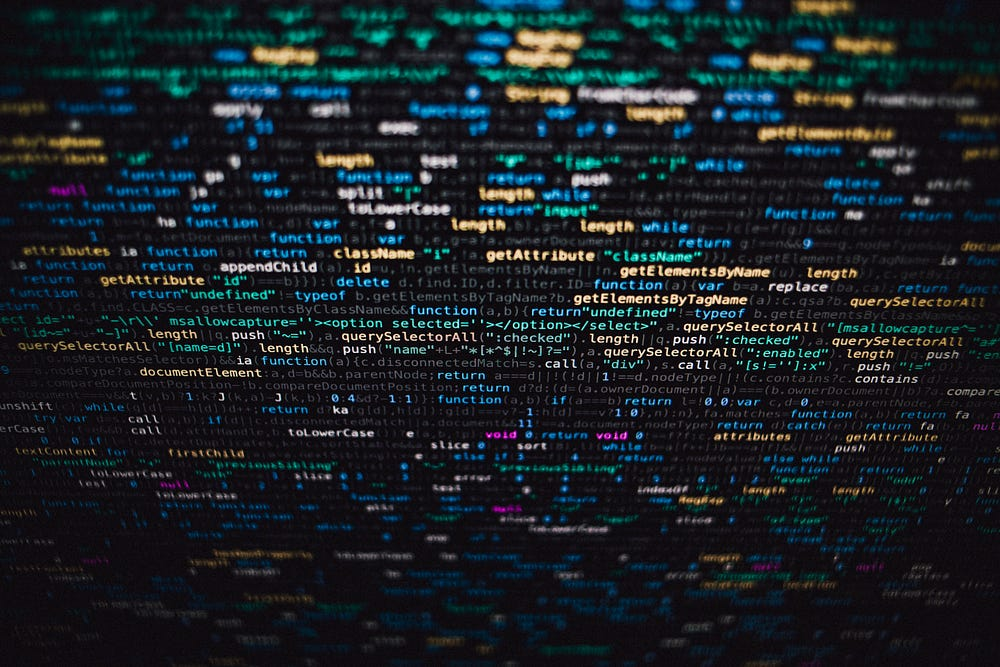# Generating new faces with Variational Autoencoders

Source: Deep Learning on Medium# Introduction

Deep generative models such as Generative Adversarial Networks (GANs) are gaining tremendous popularity, both in the industry as well as academic research. In fact, Yann LeCun, the father of the Convolutional Neural Network, described it as “the most interesting idea in the last 10 years in Machine Learning.” The idea of a computer program generating new human faces or new animals can be quite exciting. Deep generative models take a slightly different approach compared to supervised learning which we shall discuss very soon.

This quick and concise tutorial covers the basics of Deep Generative Modelling with Variational Autoencoders. I am assuming that you are fairly familiar with the concepts of Convolutional Neural Networks and representation learning. If not, I would recommend watching Andrej Karpathy’s CS231n lecture videos as they are, in my honest opinion, the best resource for learning CNNs on the internet. You can also find the lecture notes for the course here.

This example demonstrates the process of building and training a VAE using Keras to generate new faces. We shall be using the CelebFaces Attributes (CelebA) Dataset from Kaggle and Google Colab for training the VAE model.

# Generative Models

If you’re beginning to explore the field of Generative Deep Learning, a Variational Autoencoder (VAE) is ideal to kick off your journey. The VAE architecture is intuitive and simple to understand. Contrary to a discriminative model such as a CNN classifier a generative model attempts to learn the underlying distribution of the data rather than classifying the data into one of many categories. A well trained CNN classifier would be highly accurate in differentiating an image of a car from that of a house. However, this does not accomplish our objective of generating images of cars and houses.

A discriminative model learns to capture useful information from the data and utilise that information to classify a new data point into one of two or more categories. From a probabilistic perspective, a discriminative model estimates the probability 𝑝(𝑦|𝑥), where 𝑦 is the category or class and 𝑥 is the data point. It estimates the probability of a datapoint 𝑥 belonging to the category 𝑦. For example, the probability of an image being that of a car or a house.

A generative model learns the underlying distribution of the data that explains how the data was generated. In essence, it mimics the underlying distribution and allows us to sample from it to generate new data. It can be defined as estimating the probability 𝑝(𝑥), where 𝑥 is the data point. It estimates the probability of observing the data point 𝑥 in the distribution.

# Simple Autoencoder

Before delving into a Variational Autoencoder, it is crucial to analyse a simple Autoencoder.

A simple or Vanilla Autoencoder consists of two neural networks — an Encoder and a Decoder. An Encoder is responsible for converting an image into a compact lower dimensional vector (or latent vector). This latent vector is a compressed representation of the image. The Encoder, therefore maps an input from the higher dimensional input space to the lower dimensional latent space. This is similar to a CNN classifier. In a CNN classifier, this latent vector would be subsequently fed into a softmax layer to compute individual class probabilities. However in an Autoencoder, this latent vector is fed into the Decoder. The Decoder is a different neural network that tries to reconstruct the image, thereby mapping from the lower dimensional latent space to the higher dimensional output space. The Encoder and Decoder perform mappings that are exactly opposite to each other, as shown in the image img-1.

Consider the following analogy to understand this better. Imagine you’re playing a game with your friend over the phone. The rules of the game are simple. You are presented with a number of different cylinders. Your task is to describe the cylinders to your friend who will then attempt to recreate them out of modelling clay. You are forbidden from sending pictures. How will you convey this information?

Since any cylinder can be constructed with two parameters — its height and diameter, the most efficient strategy would be to estimate these two measures and convey them to your friend. Your friend, upon receiving this information can then reconstruct the cylinder. In this example, it is quite evident that you are performing the function of an Encoder by condensing visual information into two quantities. Your friend on the contrary, is performing the function of a Decoder by utilising this condensed information to recreate the cylinder.

# Housekeeping

`!pip install -U -q kaggle!mkdir -p ~/.kaggle`